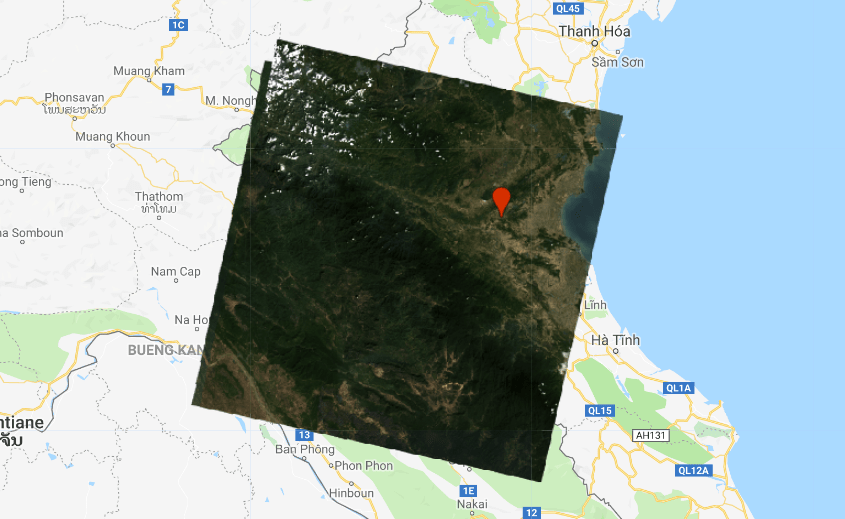# Harmonized landsat 7 & 8

Surface reflectance sensor transformation functions

Landsat-7 and Landsat-8 have different sensor characteristics. This paper compared a large number of images and presents a transform function. Applying the function will help to improve temporal continuity between the Landsat-7 ETM + and Landsat-8 OLI sensor data.

```// import the image collections
var l7 = ee.ImageCollection("LANDSAT/LE07/C01/T1_SR").filterBounds(geometry).sort("CLOUD_COVER").filterDate("1999-01-01","2003-01-01");
var l8 = ee.ImageCollection("LANDSAT/LC08/C01/T1_SR").filterBounds(geometry).sort("CLOUD_COVER");

// select first and rename the bands
var l7img = ee.Image(l7.first()).select(['B1', 'B2', 'B3', 'B4', 'B5', 'B7'],['blue', 'green', 'red', 'nir', 'swir1', 'swir2']).divide(10000);
var l8img = ee.Image(l8.first()).select(['B2','B3','B4','B5','B6','B7'],['blue', 'green', 'red', 'nir', 'swir1', 'swir2']).divide(10000);

// slope and intercept from roy et al. https://www.sciencedirect.com/science/article/pii/S0034425715302455
var slope = ee.Image.constant([0.9785, 0.9542, 0.9825, 1.0073, 1.0171, 0.9949]); // create an image of slopes per band for L8 TO L7 regression line - David Roy
var intercept = ee.Image.constant([-0.0095, -0.0016, -0.0022, -0.0021, -0.0030, 0.0029]); // create an image of y-intercepts per band for L8 TO L7 regression line - David Roy

// apply regression to landsat-8 image
var l8tol7 = l8img.subtract(intercept.divide(slope));

// display the image
```

1.Seb says:

Really helpful, thanks. Would that work also to merge landsat 5 and 8?

1.thisearthsite says:

you can not. you could adopt the method from roy et al. and apply it on l5 and l8 and use those values ..

2.bask says:

Thank you very much for your GEE script. I was just wondering why you picked reduced major axis (RMA) regression coefficients instead of ordinary least squares (OLS) regression ones tabled in Roy et al paper to harmonize L8 and L7. The authors state that the former coefficients were used throughout the paper to draw statistical conclusions thanks to the symmetrical nature of the RMA regression, and the latter one were “…provided so that the user community may apply them in their own research.”. Aren’t OLS coefficients more advisable to use?

3.Konstantin Varik says:

Hey, is there a error?
This line:
var l8tol7 = l8img.subtract(intercept.divide(slope));

Should be:
var l8tol7 = l8img.subtract(intercept).divide(slope);

4.Konstantin Varik says:

Hey, is there a error?
This line:
var l8tol7 = l8img.subtract(intercept.divide(slope));

Should be:
var l8tol7 = l8img.subtract(intercept).divide(slope);